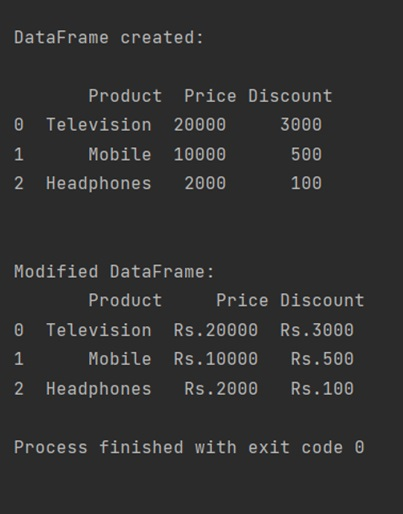# Pandas | Apply a Function to Multiple Columns of DataFrame

Pandas | Applying a function to Multiple columns: In this tutorial, we will learn how can we apply a function to multiple columns in a DataFrame with the help of example? By Pranit Sharma Last updated : April 19, 2023

## How to Apply a Function to Multiple Columns of DataFrame?

To apply a function to multiple columns of a Pandas DataFrame, you can simply use the DataFrame.apply() method by specifying the column names. The method itself takes a function as a parameter that has to be applied on the columns.

### Syntax

The following syntax shows to apply a function to multiple columns of DataFrame:

``` df[['column1','column1']].apply(anyFun);
```

Where, column1 and column2 are the column names on which we have to apply the function, and "function" has some operations that will be performed on the columns.

Let us understand with the help of an example.

## Python Program to Apply a Function to Multiple Columns of Pandas DataFrame

```# Importing pandas package
import pandas as pd

# Defining a function for modification of
# column values
def function(value):
# Adding string ='Rs.' before the value
return 'Rs.'+ value

# Creating a list of dictionary
data = {
'Price':['20000','10000','2000'],'Discount':['3000','500','100']
}

# Converting a DataFrame
df=pd.DataFrame(data)

# Display the DataFrame
print("\nDataFrame created:\n\n",df,"\n\n")

# Using the apply() method to call the function: function
df[['Price','Discount']] = df[['Price','Discount']].apply(function)

# Display modified DataFrame
print("Modified DataFrame:\n",df)
```

### Output